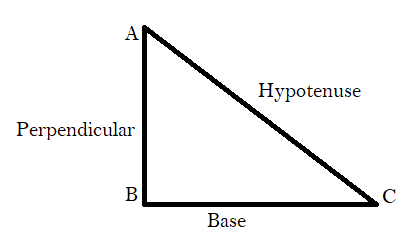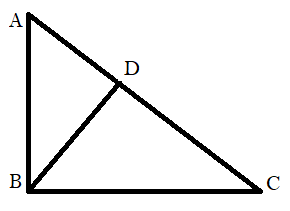Courses
Courses for Kids
Free study material
Free LIVE classes
MoreLIVE
Join Vedantu’s FREE Mastercalss

# State and prove the Pythagoras theorem.Verified
344.4k+ views
Hint: Draw a perpendicular on AC from B and use angle-angle similarity to prove the theorem.

Pythagoras theorem states that “ In a right-angled triangle, the square of the hypotenuse side is equal to the sum of squares of the other two sides”.
The sides of the right-angled triangle are called base, perpendicular and hypotenuse .According to Pythagoras theorem ,
${({\text{AC)}}^2} = {({\text{AB)}}^2}{\text{ + (BC}}{{\text{)}}^2}$
Proof:
Given, a triangle ABC in which $\angle {\text{ABC is 9}}{{\text{0}}^0}$.
Construction: Draw a perpendicular BD on AC i.e. BD $\bot$ AC.In $\Delta {\text{ABD and }}\Delta {\text{ABC }}$ we have,
$\angle {\text{BAD = }}\angle {\text{BAC }}$i.e. $\angle {\text{A}}$ is common in both triangles.
$\angle {\text{ABC = }}\angle {\text{ADB = 9}}{{\text{0}}^0}$
Therefore $\Delta {\text{ABC}} \sim \Delta {\text{ABD }}$( By AA similarity i.e. angle-angle similarity)
So,$\Rightarrow \dfrac{{{\text{AD}}}}{{{\text{AB}}}} = \dfrac{{{\text{AB}}}}{{{\text{AC}}}} \\ \Rightarrow {\text{A}}{{\text{B}}^2}{\text{ = AD}} \times {\text{AC }}...{\text{(1)}} \\$
In $\Delta {\text{BDC and }}\Delta {\text{ABC }}$ we have,
$\angle {\text{BCD = }}\angle {\text{BCA }}$i.e. $\angle {\text{C}}$ is common in both triangles.
$\angle {\text{ABC = }}\angle {\text{ADC = 9}}{{\text{0}}^0}$
Therefore $\Delta {\text{ABC}} \sim \Delta {\text{BDC }}$( By AA similarity i.e. angle-angle similarity)
So,$\Rightarrow \dfrac{{{\text{DC}}}}{{{\text{BC}}}} = \dfrac{{{\text{BC}}}}{{{\text{AC}}}} \\ \Rightarrow {\text{B}}{{\text{C}}^2}{\text{ = AC}} \times {\text{DC }}...{\text{(2)}} \\$
Adding equation (1) and (2) , we get
$\Rightarrow {\text{A}}{{\text{B}}^2}{\text{ + B}}{{\text{C}}^2}{\text{ = AD}} \times {\text{AC + AC}} \times {\text{ DC}} \\ \Rightarrow {\text{A}}{{\text{B}}^2}{\text{ + B}}{{\text{C}}^2}{\text{ = AC(AD + DC)}} \\ \Rightarrow {\text{A}}{{\text{B}}^2}{\text{ + B}}{{\text{C}}^2}{\text{ = AC(AC)}} \\ \Rightarrow {\text{A}}{{\text{B}}^2}{\text{ + B}}{{\text{C}}^2}{\text{ = A}}{{\text{C}}^2} \\$
Hence, proved.

Note: In a right angled triangle , hypotenuse is the longest side of the triangle and is opposite to the right angle i.e. ${90^0}$. By drawing a perpendicular from point B and dividing the triangle ABC into 2 parts and using angle-angle similarity to prove the Pythagoras theorem.
Last updated date: 26th Sep 2023
Total views: 344.4k
Views today: 9.44k